# Quiz 6: Percents and Their Applications in Business

Business

Let us take the number with percent 88% We have to convert the given prevents to decimals. To convert a percent to a decimal we use following two steps Step 1: Remove the percent sign Step 2: Divided by 100 From the above procedure, consider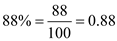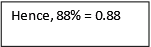Let us consider the statement A percent is a way of expressing a part of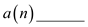We have to fill the given statement, We know that, Percents are another way of expressing quantity with relation to a whole. Percent means "per hundred" or "parts per hundred". Thus, A percent is a way of expressing a part of a hundred. Hence,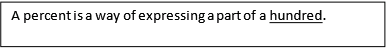Let us take the number with percent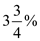We have to convert the given prevents to decimals. To convert a percent to a decimal we use following two steps Step 1: Remove the percent sign Step 2: Divided by 100 If the percent is a fraction, change the fraction to a decimal, then follow steps 1 and 2 above. From the above procedure, Consider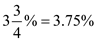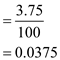There is no answer for this question

There is no answer for this question

There is no answer for this question

There is no answer for this question

There is no answer for this question

There is no answer for this question